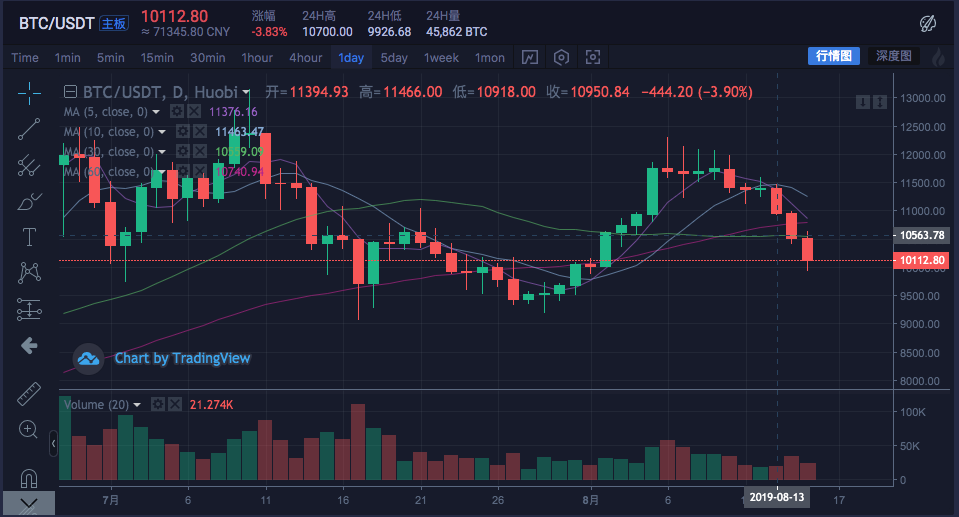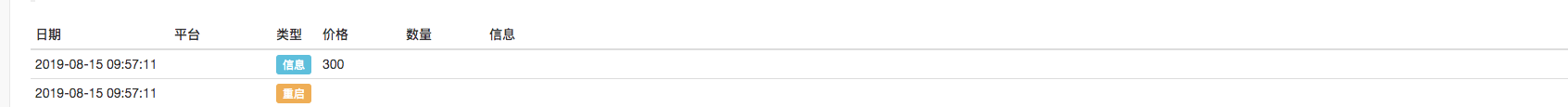[原创] 程序化交易中的K线数据处理浅谈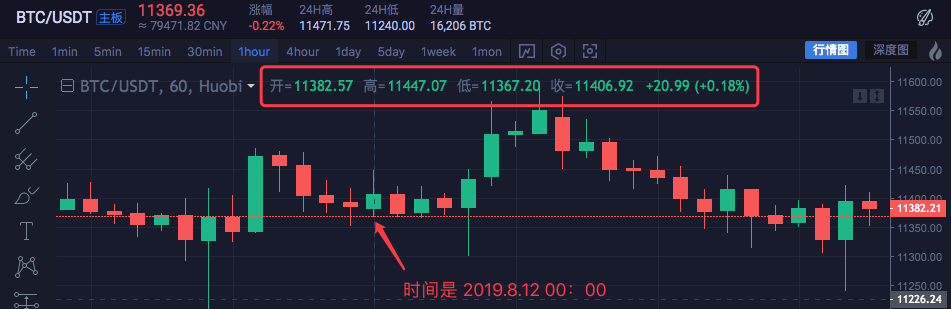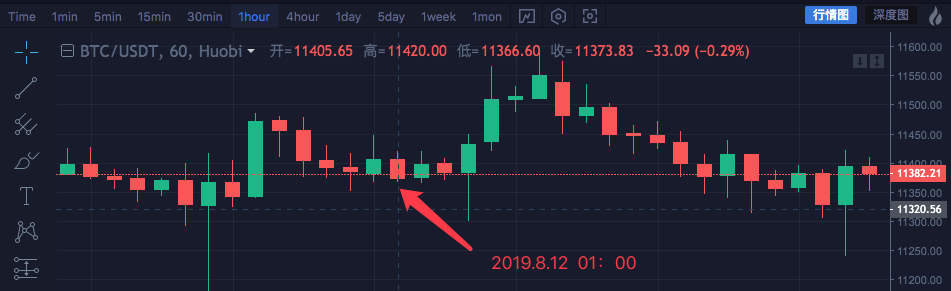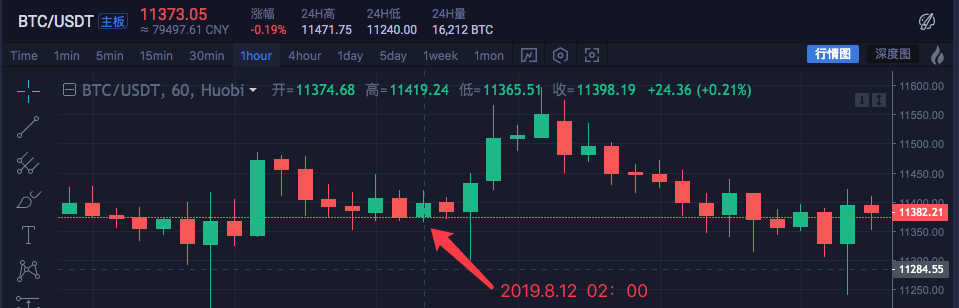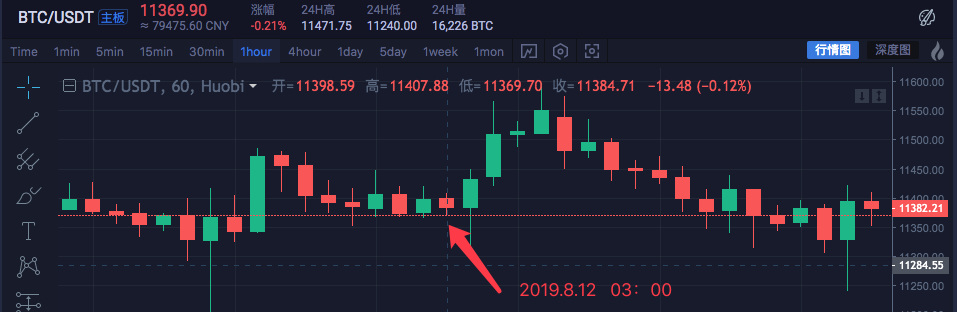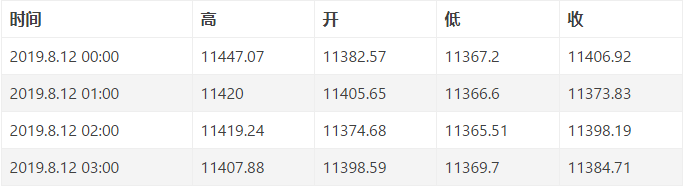• 高：11447.07
• 开：11382.57
• 低：11365.51
• 收：11384.71
• 时间：2019.8.12 00:00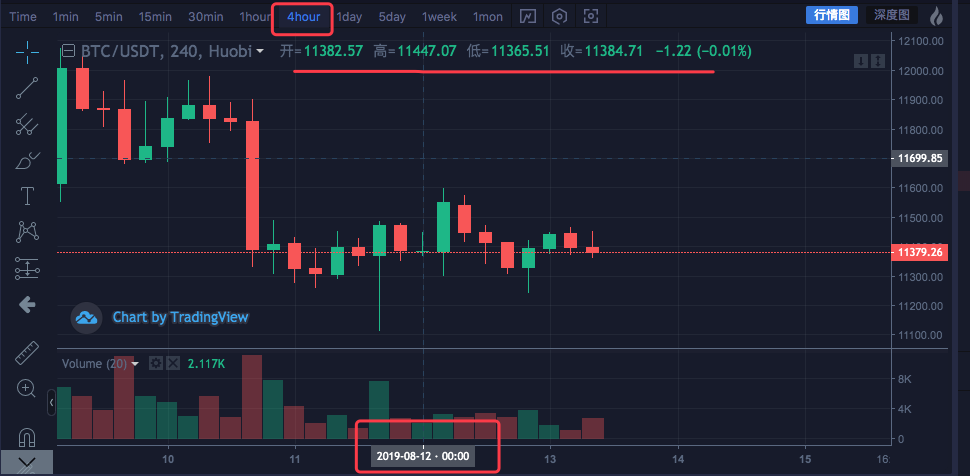function GetNewCycleRecords (sourceRecords, targetCycle) {    // K线合成函数
var ret = []

// 首先获取源K线数据的周期
if (!sourceRecords || sourceRecords.length < 2) {
return null
}
var sourceLen = sourceRecords.length
var sourceCycle = sourceRecords[sourceLen - 1].Time - sourceRecords[sourceLen - 2].Time

if (targetCycle % sourceCycle != 0) {
Log("targetCycle:", targetCycle)
Log("sourceCycle:", sourceCycle)
throw "targetCycle is not an integral multiple of sourceCycle."
}

if ((1000 * 60 * 60) % targetCycle != 0 && (1000 * 60 * 60 * 24) % targetCycle != 0) {
Log("targetCycle:", targetCycle)
Log("sourceCycle:", sourceCycle)
Log((1000 * 60 * 60) % targetCycle, (1000 * 60 * 60 * 24) % targetCycle)
throw "targetCycle cannot complete the cycle."
}

var multiple = targetCycle / sourceCycle

var isBegin = false
var count = 0
var high = 0
var low = 0
var open = 0
var close = 0
var time = 0
var vol = 0
for (var i = 0 ; i < sourceLen ; i++) {
// 获取 时区偏移数值
var d = new Date()
var n = d.getTimezoneOffset()

if (((1000 * 60 * 60 * 24) - sourceRecords[i].Time % (1000 * 60 * 60 * 24) + (n * 1000 * 60)) % targetCycle == 0) {
isBegin = true
}

if (isBegin) {
if (count == 0) {
high = sourceRecords[i].High
low = sourceRecords[i].Low
open = sourceRecords[i].Open
close = sourceRecords[i].Close
time = sourceRecords[i].Time
vol = sourceRecords[i].Volume

count++
} else if (count < multiple) {
high = Math.max(high, sourceRecords[i].High)
low = Math.min(low, sourceRecords[i].Low)
close = sourceRecords[i].Close
vol += sourceRecords[i].Volume

count++
}

if (count == multiple || i == sourceLen - 1) {
ret.push({
High : high,
Low : low,
Open : open,
Close : close,
Time : time,
Volume : vol,
})
count = 0
}
}
}

return ret
}

// 测试
function main () {
while (true) {
var r = exchange.GetRecords()                           // 原始数据，作为合成K线的基础K线数据，例如要合成4小时K线，可以用1小时K线作为原始数据。
var r2 = GetNewCycleRecords(r, 1000 * 60 * 60 * 4)      // 通过 GetNewCycleRecords 函数 传入 原始K线数据 r , 和目标周期， 1000 * 60 * 60 * 4 即 目标合成的周期 是4小时K线数据。

\$.PlotRecords(r2, "r2")                                 // 策略类库栏 可以勾选画线类库，调用 \$.PlotRecords 画线类库 导出函数 画图。
Sleep(1000)                                             // 每次循环间隔 1000 毫秒，防止访问K线接口获取数据过于频繁，导致交易所限制。
}
}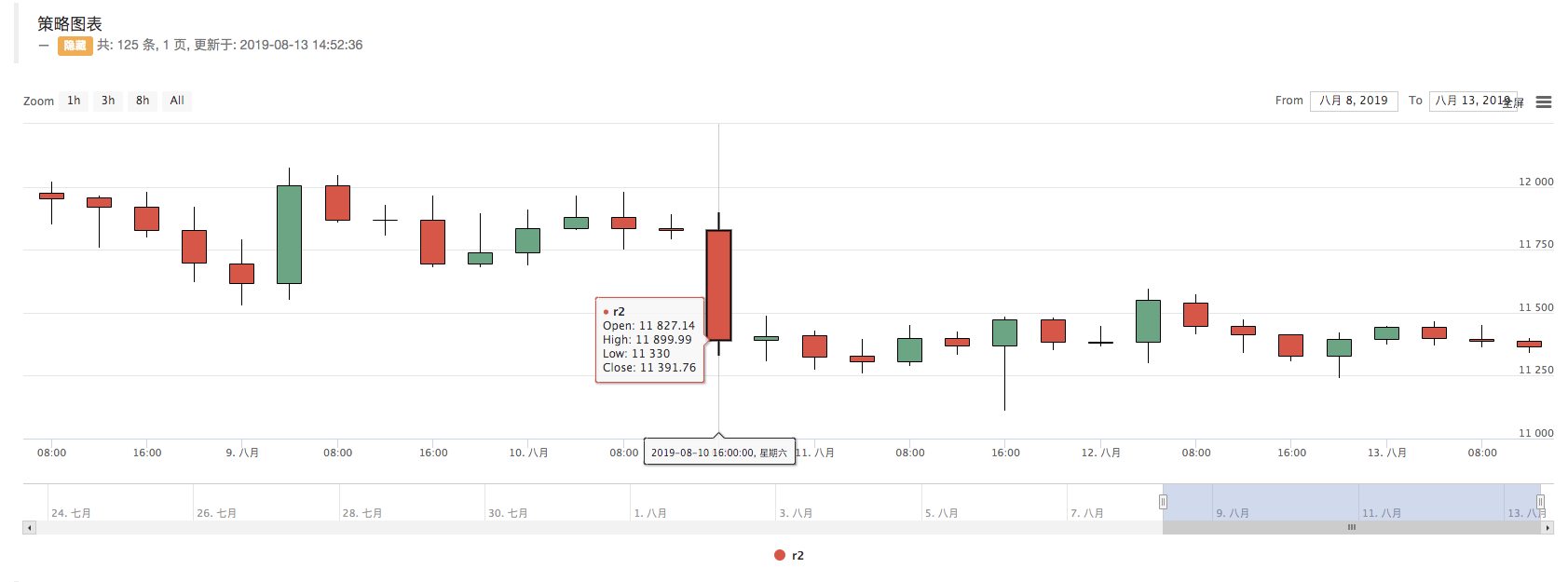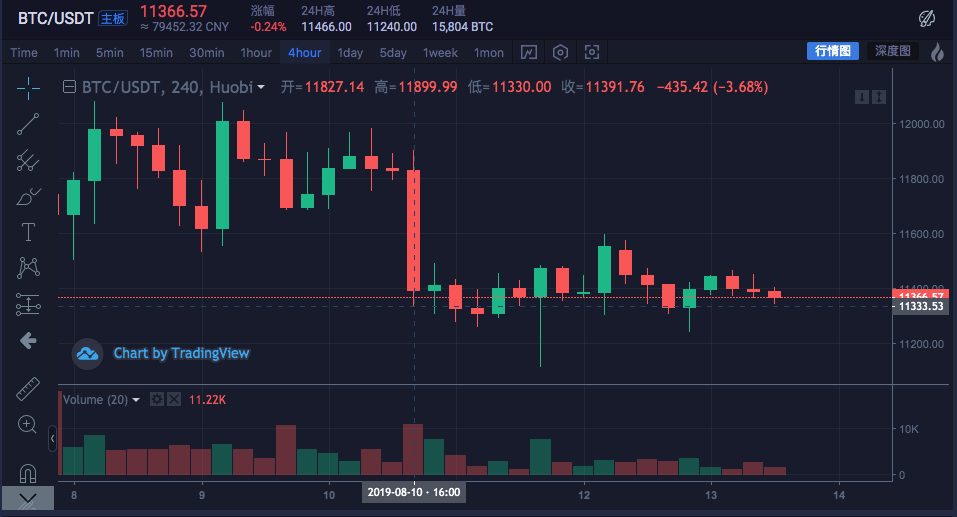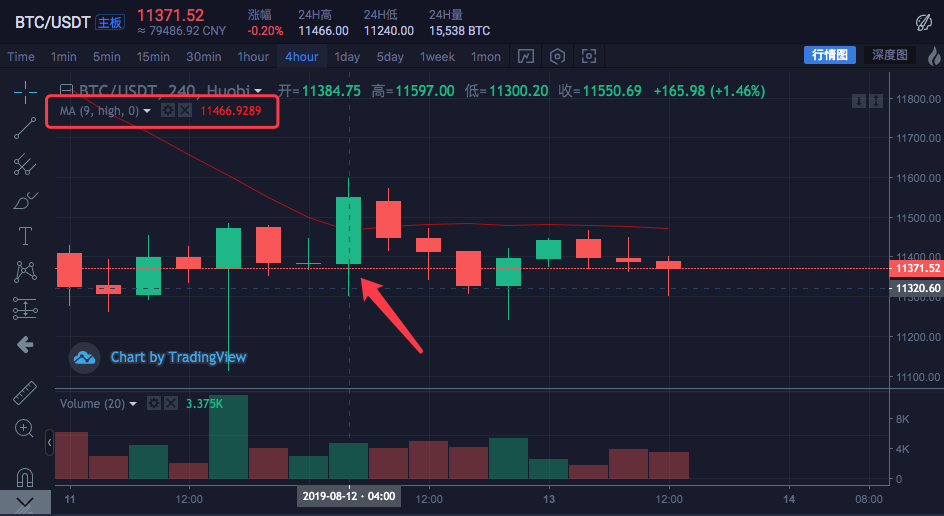K线周期是4小时，在交易所图表上，已经设置好了一条均线，均线周期参数为9。并且设置计算的数据源是每根Bar的最高价。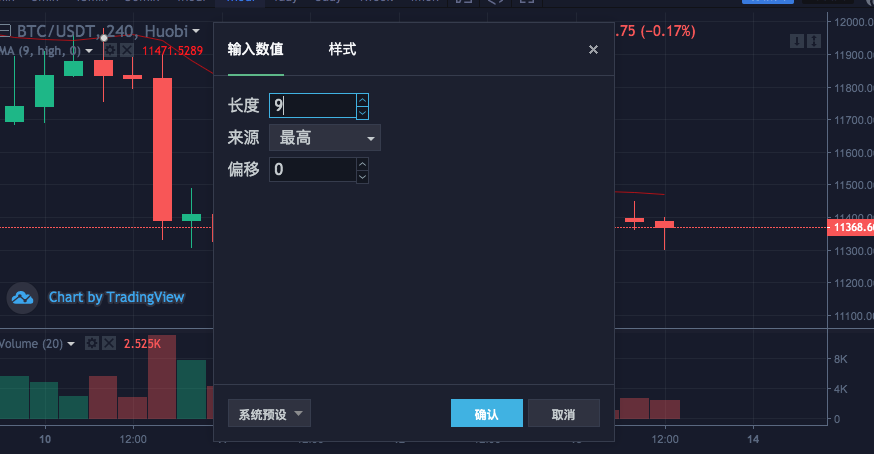var highs = []
for (var i = 0 ; i < r2.length ; i++) {
highs.push(r2[i].High)
}

function main () {
while (true) {
var r = exchange.GetRecords()
var r2 = GetNewCycleRecords(r, 1000 * 60 * 60 * 4)
if (!r2) {
continue
}

\$.PlotRecords(r2, "r2")                                               // 画出K线

var highs = []
for (var i = 0 ; i < r2.length ; i++) {
highs.push(r2[i].High)
}

var ma = talib.MA(highs, 9)                                           // 用均线指标函数 talib.MA 计算 均线指标
\$.PlotLine("high_MA9", ma[ma.length - 2], r2[r2.length - 2].Time)     // 使用画线类库把均线指标画在图表上

Sleep(1000)
}
}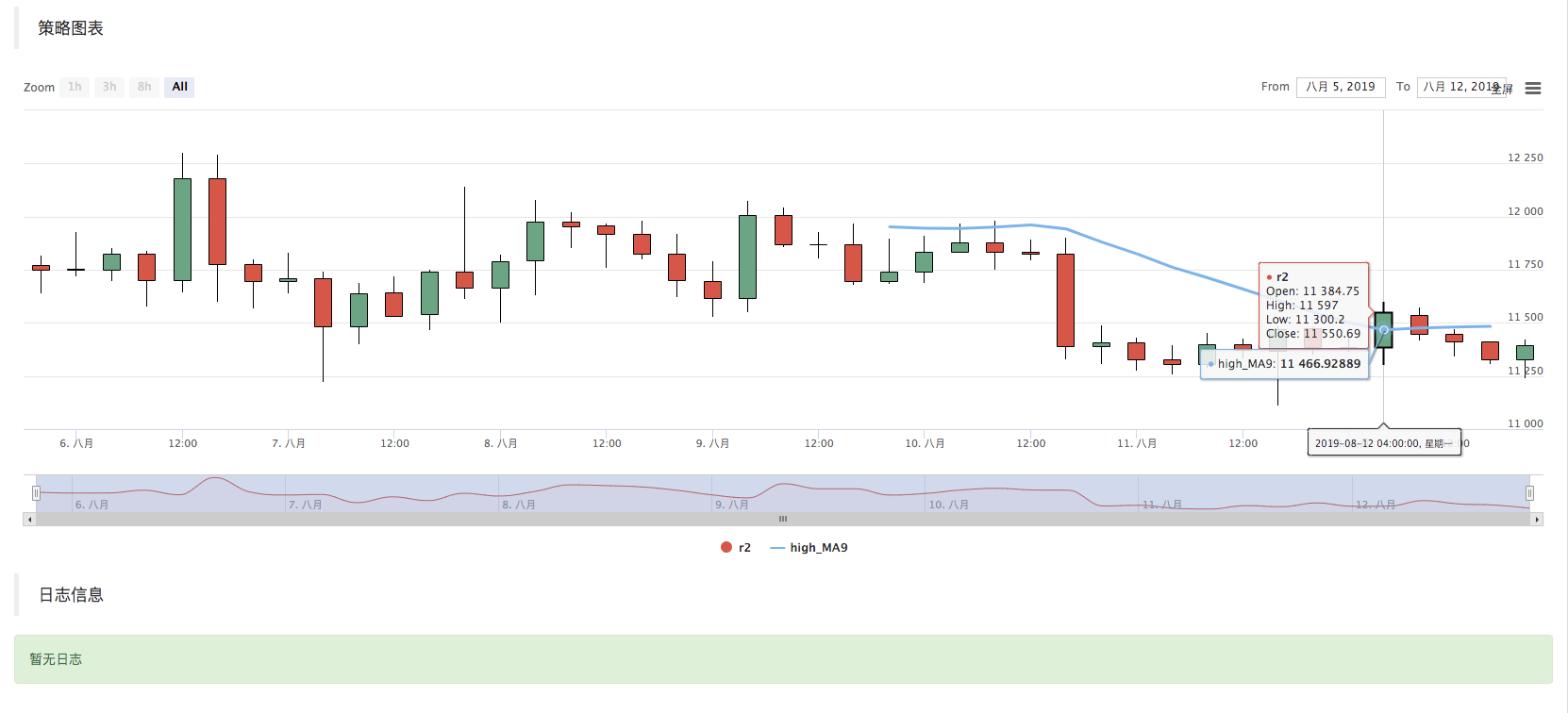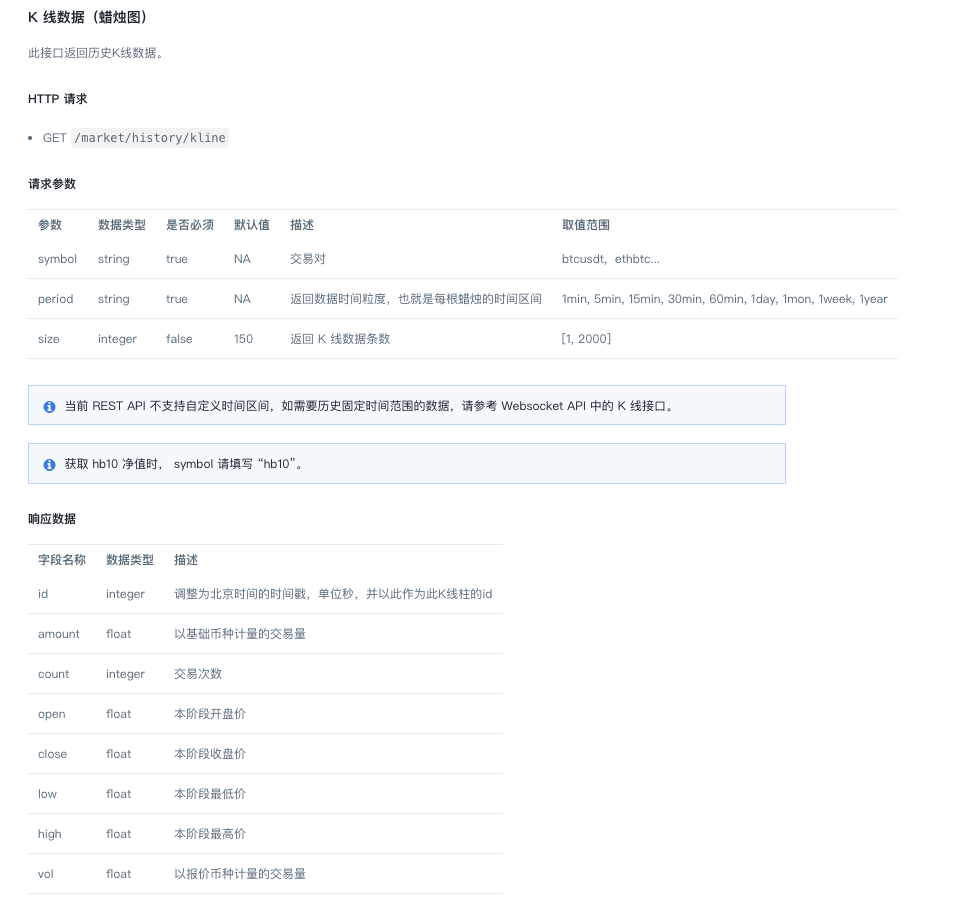https://api.huobi.pro/market/history/kline?period=1day&size=200&symbol=btcusdt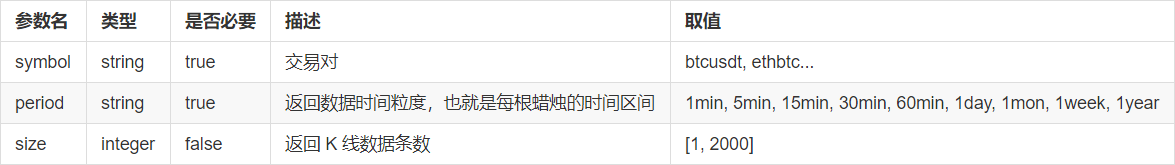function GetRecords_Huobi (period, size, symbol) {
var url = "https://api.huobi.pro/market/history/kline?" + "period=" + period + "&size=" + size + "&symbol=" + symbol
var ret = HttpQuery(url)

try {
var jsonData = JSON.parse(ret)
var records = []
for (var i = jsonData.data.length - 1; i >= 0 ; i--) {
records.push({
Time : jsonData.data[i].id * 1000,
High : jsonData.data[i].high,
Open : jsonData.data[i].open,
Low : jsonData.data[i].low,
Close : jsonData.data[i].close,
Volume : jsonData.data[i].vol,
})
}
return records
} catch (e) {
Log(e)
}
}

function main() {
var records = GetRecords_Huobi("1day", "300", "btcusdt")
Log(records.length)
\$.PlotRecords(records, "K")
}